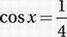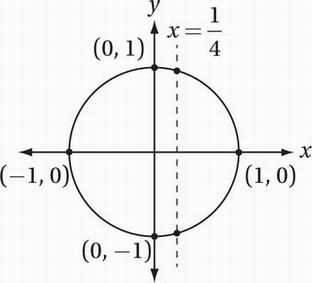# SAT Math Multiple Choice Question 627: Answer and Explanation

### Test Information

Question: 627

12. How many solutions to the equation 4 cos x = 1 lie between x = 0 and x = 3π?

• A. Two
• B. Three
• C. Four
• D. Six

Explanation:

B

Special Topics (trigonometry) MEDIUM-HARD

In order to solve this without a calculator, we need to know how to analyze this problem in terms of the unit circle. First, let's solve for cos x:

4 cos x = 1

Divide by 4:What does the mean in terms of the unit circle? Recall from Chapter 10, Lesson 9, that the cosine of any angle corresponds to the x-coordinate of the corresponding point for that angle on the unit circle:Notice that there are exactly two points on the unit circle that have an x-coordinate of 1/4. Now let's think about the angle. We are told that x goes from 0 to 3π. Remember that a full trip around the circle is 2π radians; therefore, a journey from x = 0 to x = 3π is 1.5 trips around the circle counterclockwise starting from the positive x-axis. If you trace with your finger 1.5 times around the circle starting from the point (1, 0), you'll hit our "points of interest" exactly three times.